# Prof. Bryan Caplan

bcaplan@gmu.edu

http://www.gmu.edu/departments/economics/bcaplan

Econ 816

Spring, 2000

Part 1: Mathematical Problems

1.  Romer, problem 9.8.

a.  Since this is an RE model without exogenous uncertainty, the fact that expected inflation pe=b/a implies that actual inflation p=b/a.  Therefore,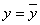.

b.  The AS function in the intial period will be:The 1-period OF is therefore:Differentiating: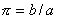, so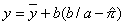Plugging into the lifetime OF yields: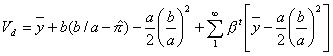Whereas if the policymaker did not defect, lifetime OF would be:c.  Find when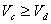: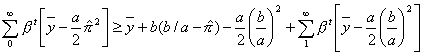Cancelling and simplifying: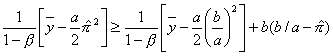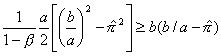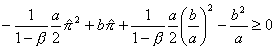This leaves us with a quadratic equation in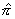.  Solve using the quadratic formula, it can be seen that the above inequality holds if: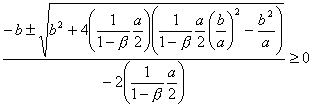Simplifying: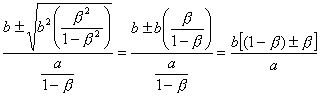The two critical values are thus: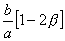and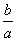.

Cooperation will then be possible so long as: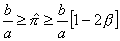.

If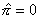, it cannot be larger than b/a, so equilibrium will exist so long as: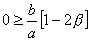, implying that.

2.  Romer, problem 9.9.

In all three problems, the cooperation utility remains:a.  Defection will only pay if one can defect one period, take the punishment for one period, and be better off than if one had cooperated.  So compare: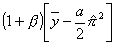to: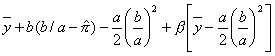Simplifying: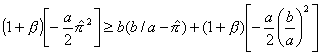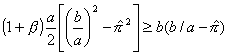Again solving the quadratic equation for the critical values (and simplifying!):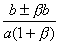, so the critical values areand.

Cooperation will then be possible so long as: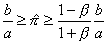.

b. This implies a two-period cost of defection.  For convenience, define.  Then compare: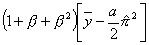to: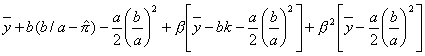So in equilibrium: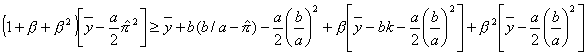Simplifying: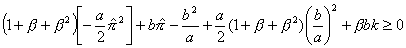Applying the quadratic formula yields the following mess: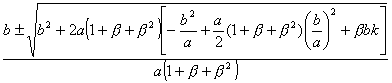Which shows that with extreme punishments, you can get cooperation in more general circumstances than you can otherwise.

c.  This is always an equilibrium; in each period, the policy-maker is optimizing relative to the public's actions, and vice versa.

3.  Romer, problem 9.16.

ln(Mt/Pt)=a-bi+ln Yt, so:

Mt/Pt=ea-biYt

i=r+pe

The percent change in the money supply plus the inflation rate must equal the real rate of growth, so:

p=gM-gY

i=r+gM-gY

Plugging into the equation for real balances:

Mt/Pt=exp(a)exp(-b[r+gM-gY])Yt

Seigniorage equals the change in real balances: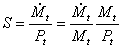Plugging in:

S=gM*exp(a)exp(-b[r+gM-gY])Yt

Then just maximize S wrt gM, getting:

[exp(a)exp(-b[r+gM-gY])Yt]-bgM[exp(a)exp(-b[r+gM-gY])Yt]=0

Thus:

[exp(a)exp(-b[r+gM-gY])Yt][1-bgM]=0

The first term in brackets is always positive, so the only solution is given by:

[1-bgM]=0

Implying gM*=1/b

4.  Romer, problem 9.17.

a. As in the last problem,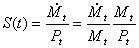.

Define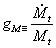and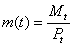.  Then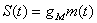Next, note that inflation is just the difference between nominal money growth and real money growth: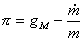Let G be the amount of seigniorage the government spends: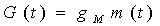, soThe money demand equation can be used to compute: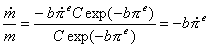Plugging into the equation for inflation: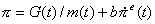Now plug the above into the equation for the change in expected inflation:, so: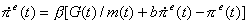Re-arranging terms: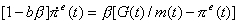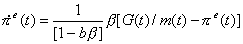b.  In this case, you essentially get a hyperinflation - every period to have to make inflation higher to get more than S* in revenue.

c.  Now in the steady state, expected inflation equals actual inflation equals the rate of money growth.